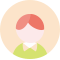Hi, 欢迎访问门门•IOS
•Android

• 极墨
• 乐乐喵
• 科威顿
• 米妮
• 利瑞芬
• 宝宠派
• 梦雷宠
• 鹿凌青
• 金娘子
• 短云
• 搭啵兔
• 彝家有礼
• 嬉皮狗
• 华幻
• Truelove
• 悠乐宠

• 狗狗
• 猫猫
• 猫狗通用
• 金丝熊
• 松鼠
• 龙猫
• 仓鼠
• 蜜袋鼯
• 巴西龟
• 兔兔通用
• 仓鼠通用
• 鼠类通用
• 蝾螈
• 犬用

• 胸背带
• 项圈
• 牵引
• 牵引带
• 保护盖
• 普通牵引绳
• 拖车绳
• 训导玩具
• 玩具
• 厕所
• 自动牵引绳
• 胸背牵引带套装
• 日常用品
• 果蔬干
• 可伸缩牵引类
• 浴室
• 绳子
• 挂牌
• 伸缩链
• 棉衣
• 益生菌
• 垫料
• 背心

• 原色
• 黑色
• 红色
• 混色
• 蓝色
• 粉色
• 白色
• 棕色
• 橙色
• 经典黑色沙漠迷彩色
• 黄色
• 珠光白
• 粉红色
• 卡其色
• 灰色
• 橘色
• 绿色
• l号带状8米2件套
• 单胸背带
• 小佩胸背+
• 红牵引
• 胸背带
• 活力橙套装

• 通用
• 小型宠物
• 中型宠物

• 金属
• 布料
• 涤纶
• 棉麻
• 帆布
• 棉麻+帆布+棉+布
• PU
• 亚麻
• 塑料
• 聚酯纤维
• TPR
• 铝合金+布
• 人造纤维
• 尼龙
• ABS
• 合金
• 棉麻+帆布+塑料+布
• 钛钢
• 植物纤维
• 不锈钢
• 钢丝

• 老龄犬
• 繁育犬
• 1岁
• 14岁以上
• 3岁
• 0-3个月
• 3岁以上
• 6-12个月
• 2-4岁
• 1-5岁
• 2岁/18个月
• 8个月
• 所有
• 1-3岁
• 3岁/2岁/18个月
• 幼宠
• 最小1岁

• 防走失手环
• 防走失背包
• 防走失背带
• 手提包
• 自动伸缩夜间反光
• 竞技款
• 十字链
• xs
• 双肩包
• m
• 斜挎包
• 背负式
• 5m
• l-15-30斤
• 不限
• 分体式
• 升级款灰色格子铃铛
• 红天使
• 3m
• 8m
• m码
• s
• s斤
• 两脚衣

-

• 1
• 2
• 3
• 4
• 5
• 6
• 7
• 8
• 9
• 10